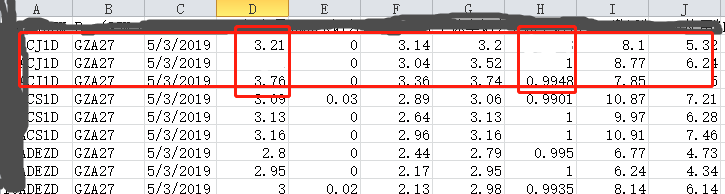python 处理csv或excel文件时遇到空白单元格怎么处理。``````a1=[1,3,2.3,3]
a2=[5,2,3,5.5]
suma12=[c+d for (c,d) in zip(a1,a2)]
print (suma12)
#output：[6, 5, 5.3, 8.5]
``````

``````a1=[1,,2.3,3,,3'3]
a2=[5,2,,5.5]
suma12=[b+c for (b,c) in zip(a1,a2)]
print (suma12)
#SyntaxError: invalid syntax
``````Tyche_BO 回复操作员马善福（贵阳专业挖机）: 很好笑

1个回答